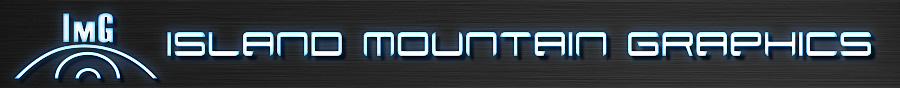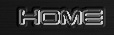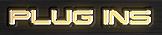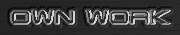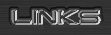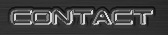Deutsche Version

### photoshop plugins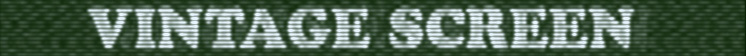## examples

Here are some examples made with the "vintage screen" plugin.
The image size is 600x400, no other processing was done. Only the plugin with the parameters shown below. The parameter that dominates this special look are underlined.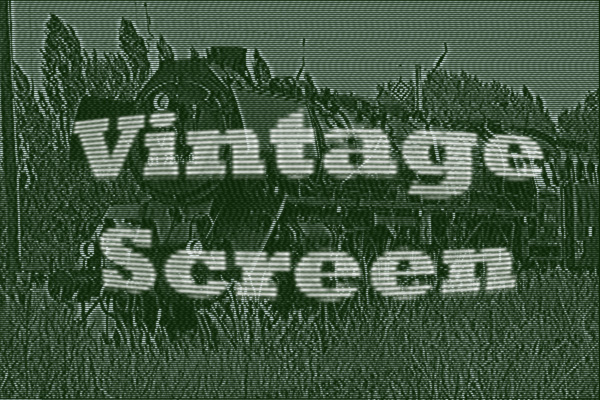This was made with two layers. The lower layer was a normal image layer. The upper layer was a layer with rasterized font. White on black.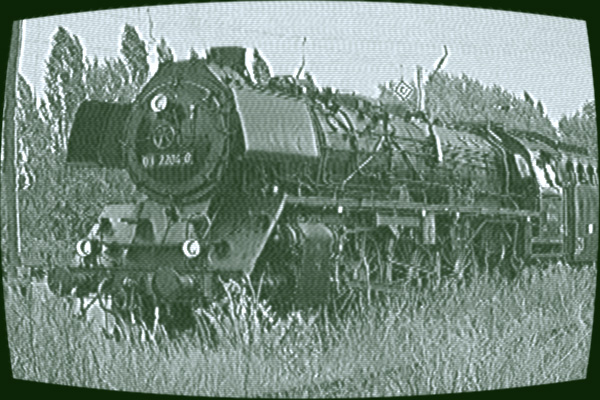lines = 2 interlaced = 0 ghost distance = ---- ghost level = 0 noise = 0.13 tuning = 0.18 sync = 0.01 black level = 0.6 video level = 1.01 focus = 0.45 glow = 0.1 screen bulge = 0.55 exposure time = --- exposure offset = ---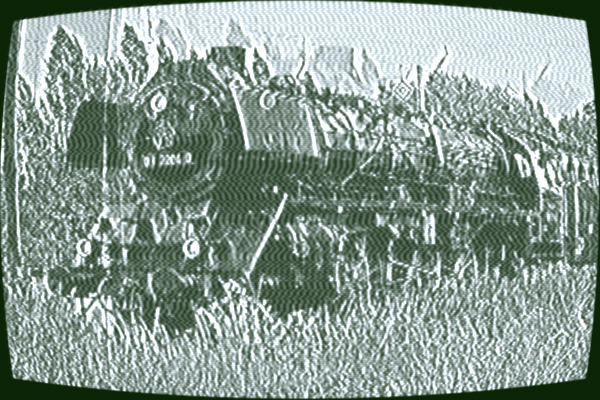lines = 2 interlaced = 0 ghost distance = 9.29 ghost level = 0.21 noise = 0.13 tuning = 0.48 sync = 0 black level = 0 video level = 1.23 focus = 0.75 glow = 0.1 screen bulge = 0.59 exposure time = --- exposure offset = ---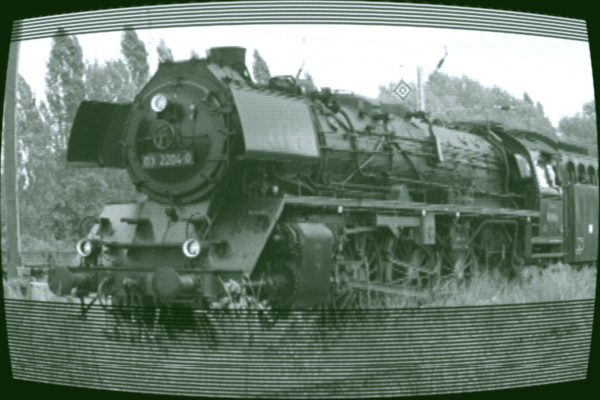lines = 1 interlaced = 0 ghost distance = --- ghost level = 0 noise = 0 tuning = 0.02 sync = 0 black level = 0.06 video level = 1.09 focus = 0.75 glow = 0.1 screen bulge = 0.59 exposure time = 1/30s exposure offset = 0.58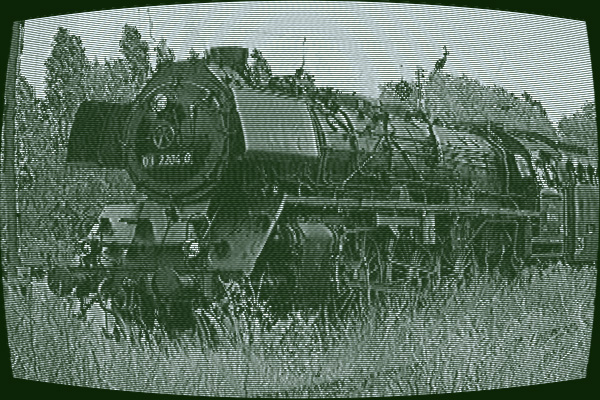lines = 2 interlaced = 0 ghost distance = 5.29 ghost level = 0.08 noise = 0.07 tuning = 0.12 sync = 0.01 black level = 0.1 video level = 1.5 focus = 0.15 glow = 0.1 screen bulge = 0.59 exposure time = --- exposure offset = ---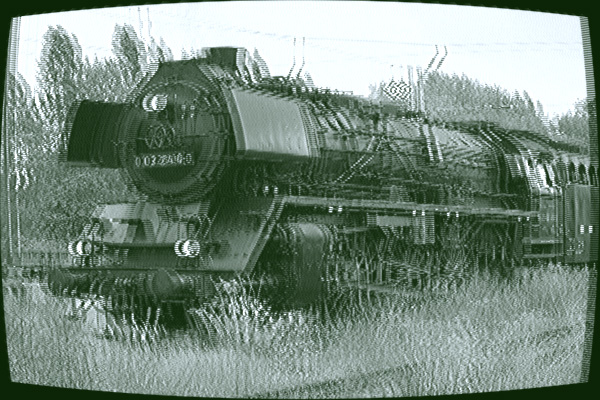lines = 1 interlaced = 0.33 ghost distance = --- ghost level = 0 noise = 0.04 tuning = 0.1 sync = 0 black level = 0 video level = 1.18 focus = 0.75 glow = 0.1 screen bulge = 0.55 exposure time = --- exposure offset = ---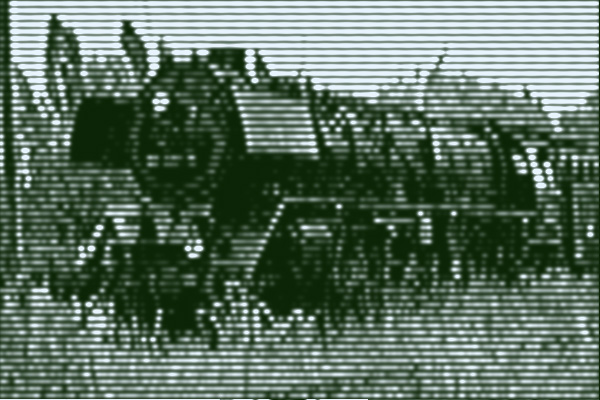lines = 7 interlaced = 0 ghost distance = --- ghost level = 0 noise = 0.02 tuning = 0.1 sync = 0 black level = 0 video level = 1.96 focus = 0.45 glow = 0.1 screen bulge = 0.55 exposure time = --- exposure offset = ---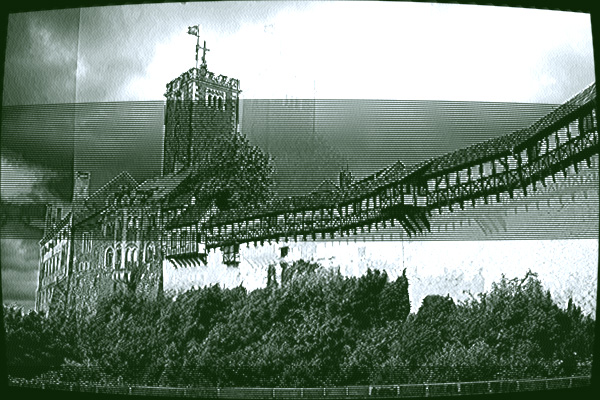lines = 1 interlaced = 0 ghost distance = 6.59 ghost level = 0.18 noise = 0.01 tuning = 0.1 sync = 0 black level = 0 video level = 1.58 focus = 0.45 glow = 0.1 screen bulge = 0.49 exposure time = 1/30s exposure offset = 0.32

This one is an example of little abuse. No low "vintage" quality processing was done. Only coloration by using colormode "photoshop" and a yellow (241, 213, 99) as photoshop's forground color and a dark blue (1, 25, 86) as background color. All other parameter are set to neutral position.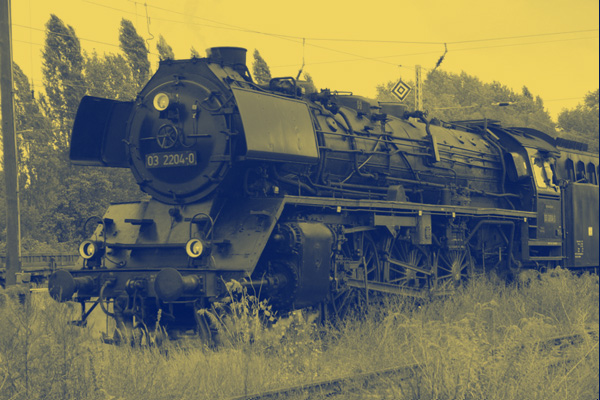lines = 1 interlaced = 0 ghost distance = --- ghost level = 0 noise = 0 tuning = 0 sync = 0 black level = 0 video level = 1 focus = 0.5 glow = 0 screen bulge = 0 exposure time = --- exposure offset = ---

 copyright 2007 by Thomas Thiele last actualisation 5th August 2007